# Generalized stacking faults

The concept of a generalized stacking fault

(or γ-surface) was introduced in the 1960's by Vitek on bcc metals . The misfit energy across a shear plane is derived by displacing the two half of a crystal of a given quantity in that plane and then by allowing atoms to relax normal to the shear plane. The process is then repeated so as to cover any shear direction in a given plane. For each energy obtained during a displacement, we remove the energy of the crystal without displacement. The energy difference is finally divided by the surface of the plane. Then the entire energy surface is generated. The example is 2D (displacements along one direction only in a given plane) for easiest visualisation, the result is a gamma-line along  in (100).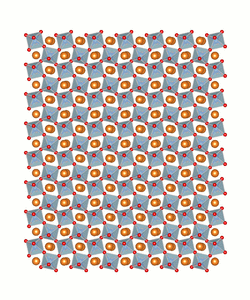Supercell and successive steps for gamma-line calculation. A rigid body shear is applied (at 30 GPa here) on Mg-Pv along  and in (100).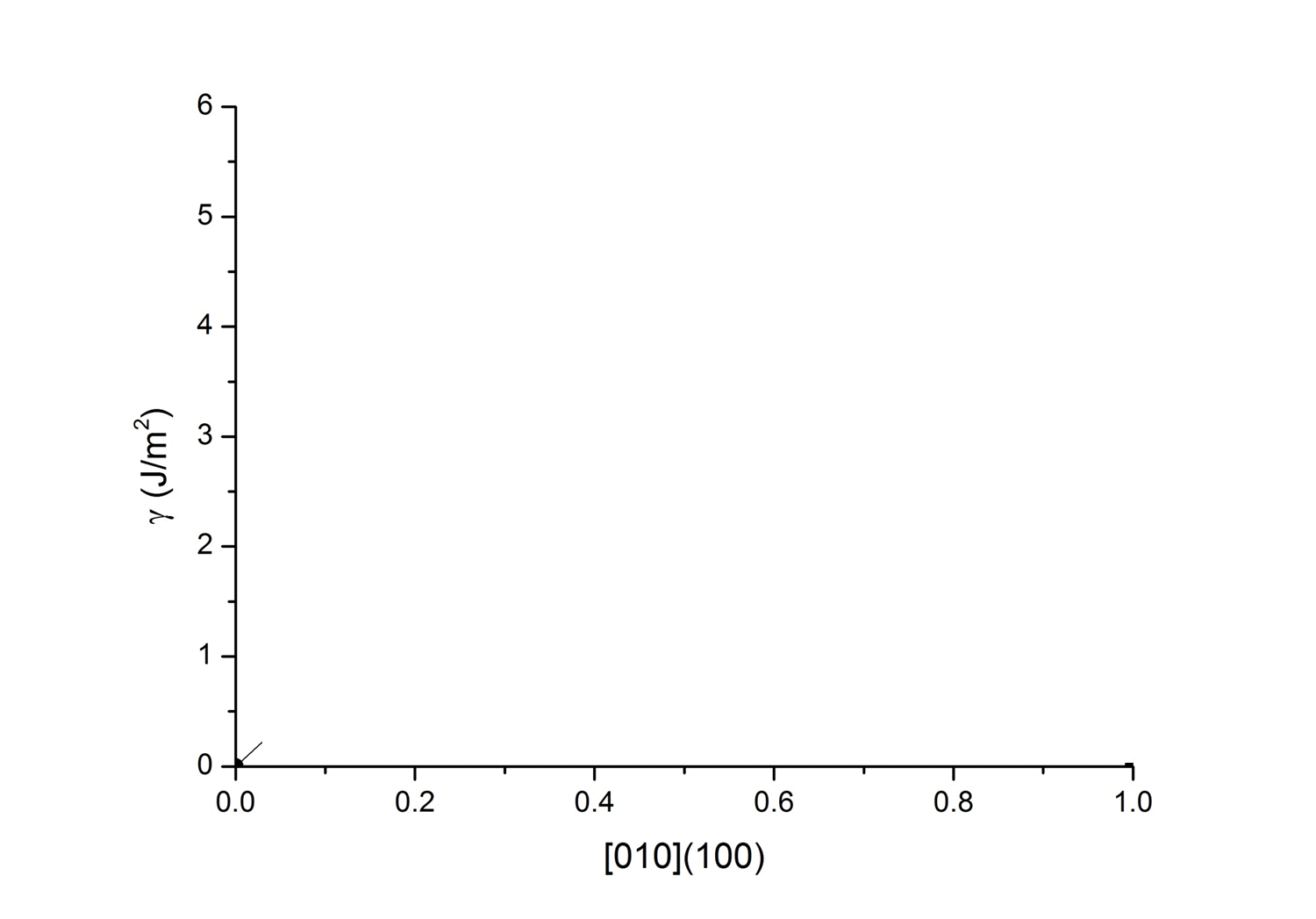Corresponding gamma- line along  in (100), at 30 GPa.

Examples: γ-surfaces in MgSiO3 perovskite

are calculated by atomistic potential calculations. The latters are performed using Buckingham  pairwise potentials as implemented in the LAMMPS code. We present here the gamma-surfaces for (100), (010), (001), (110), (101) and (011)  obtained at 30 GPa.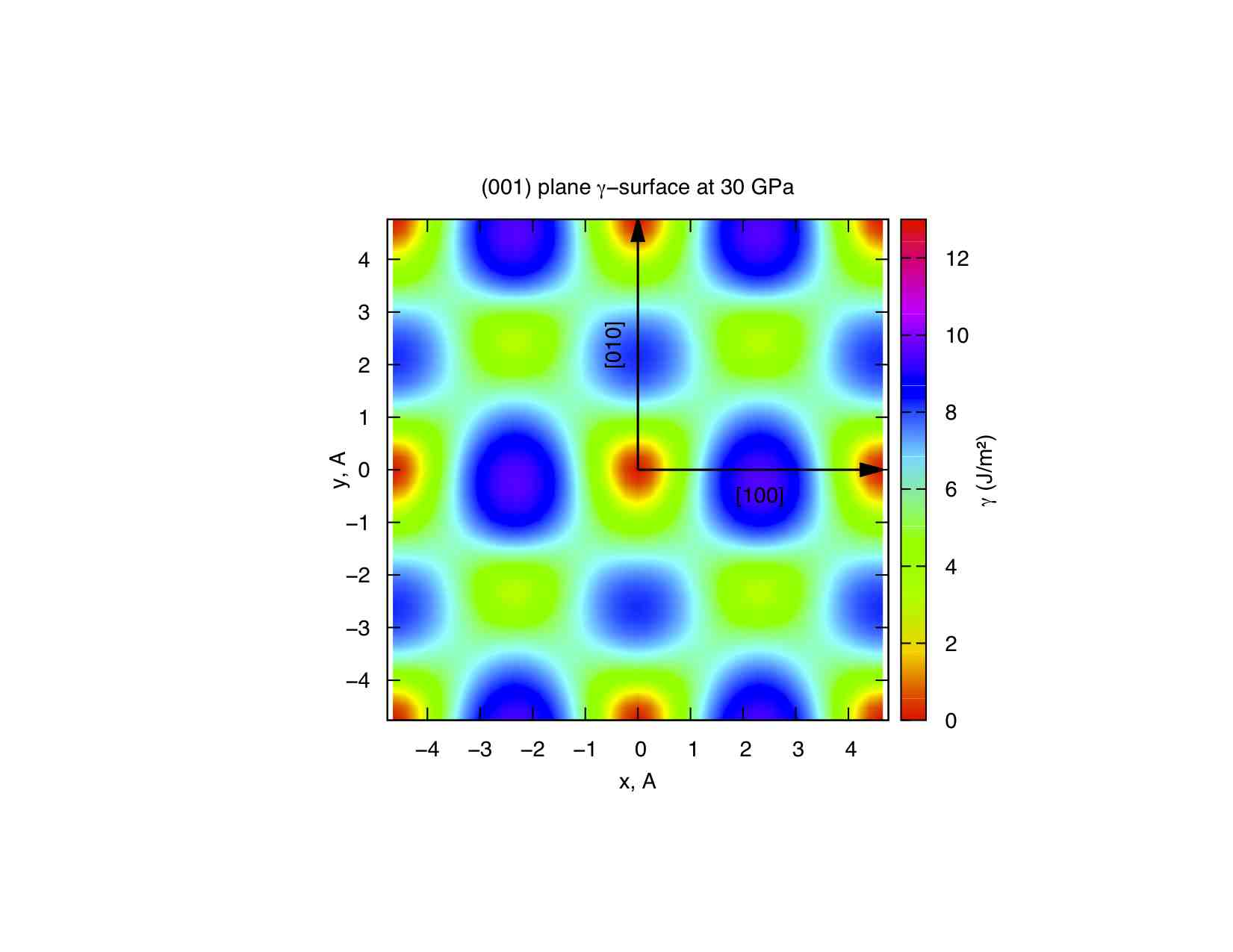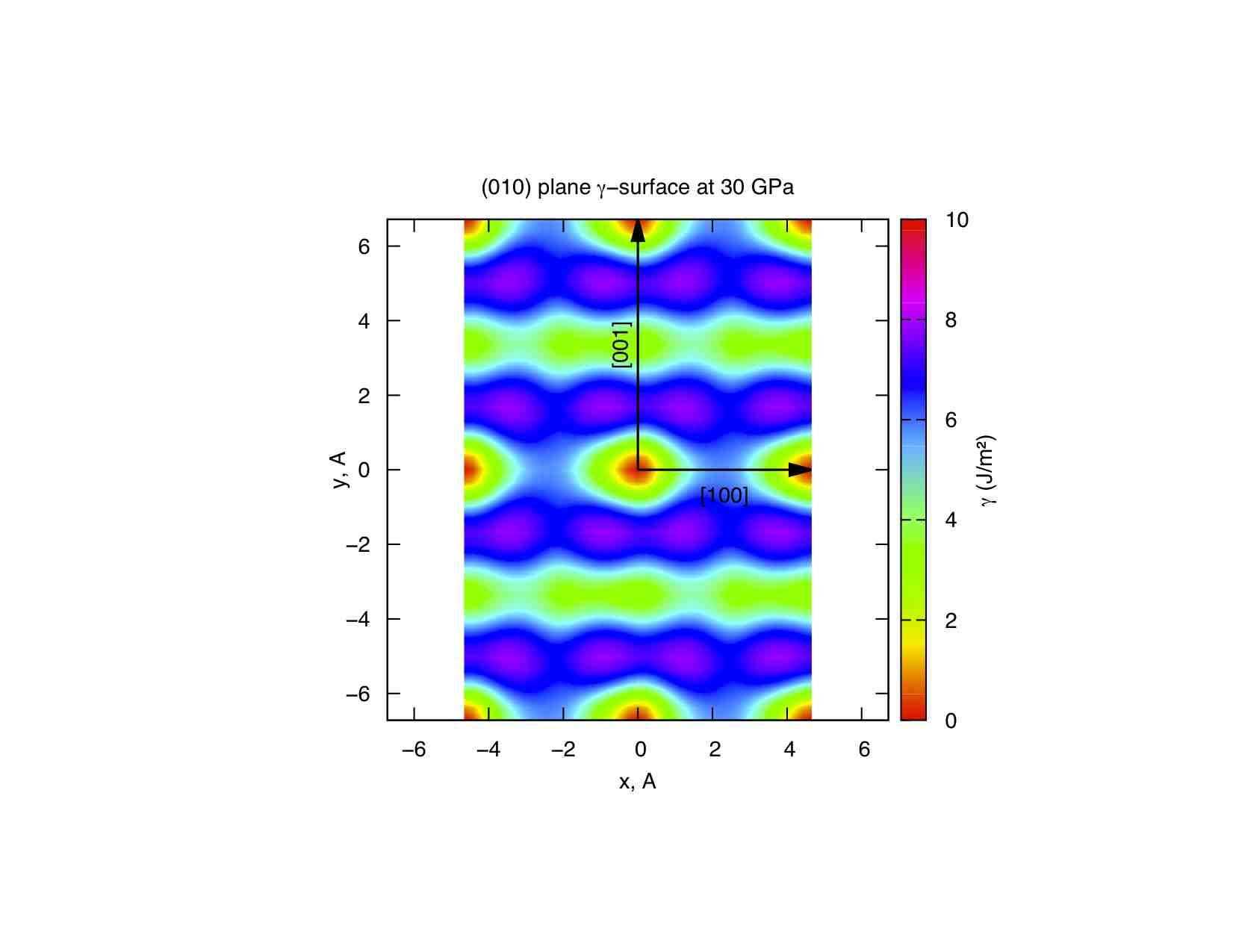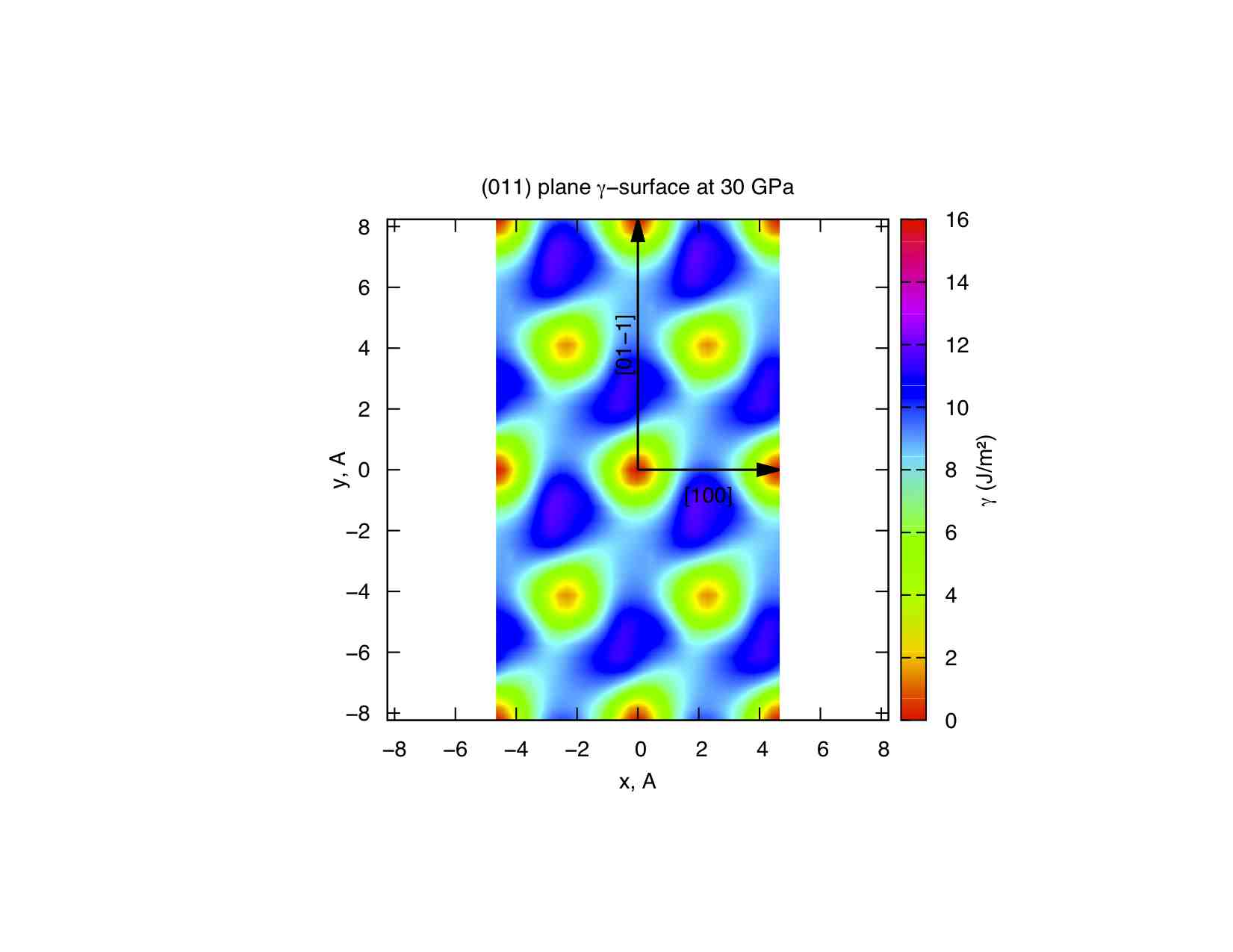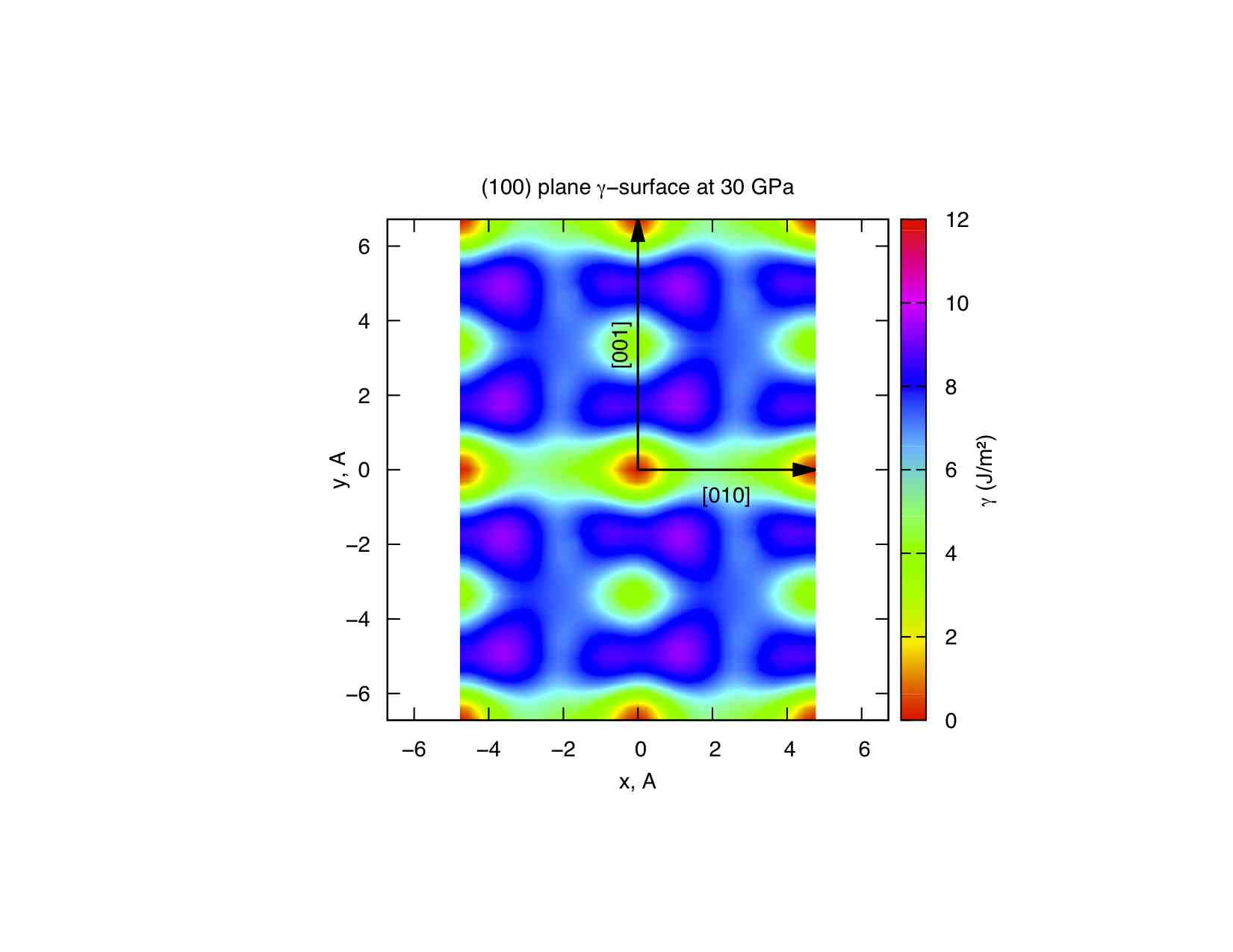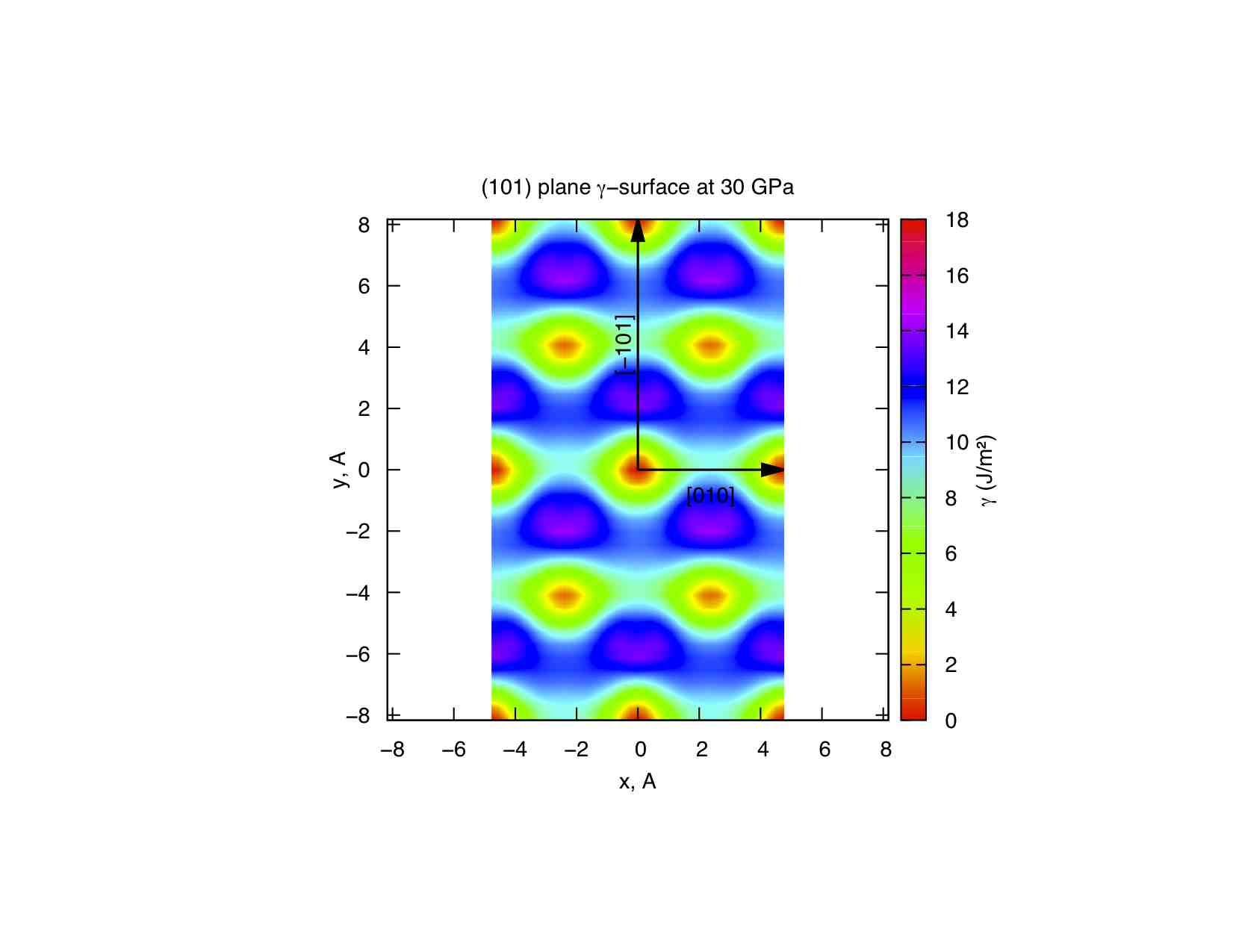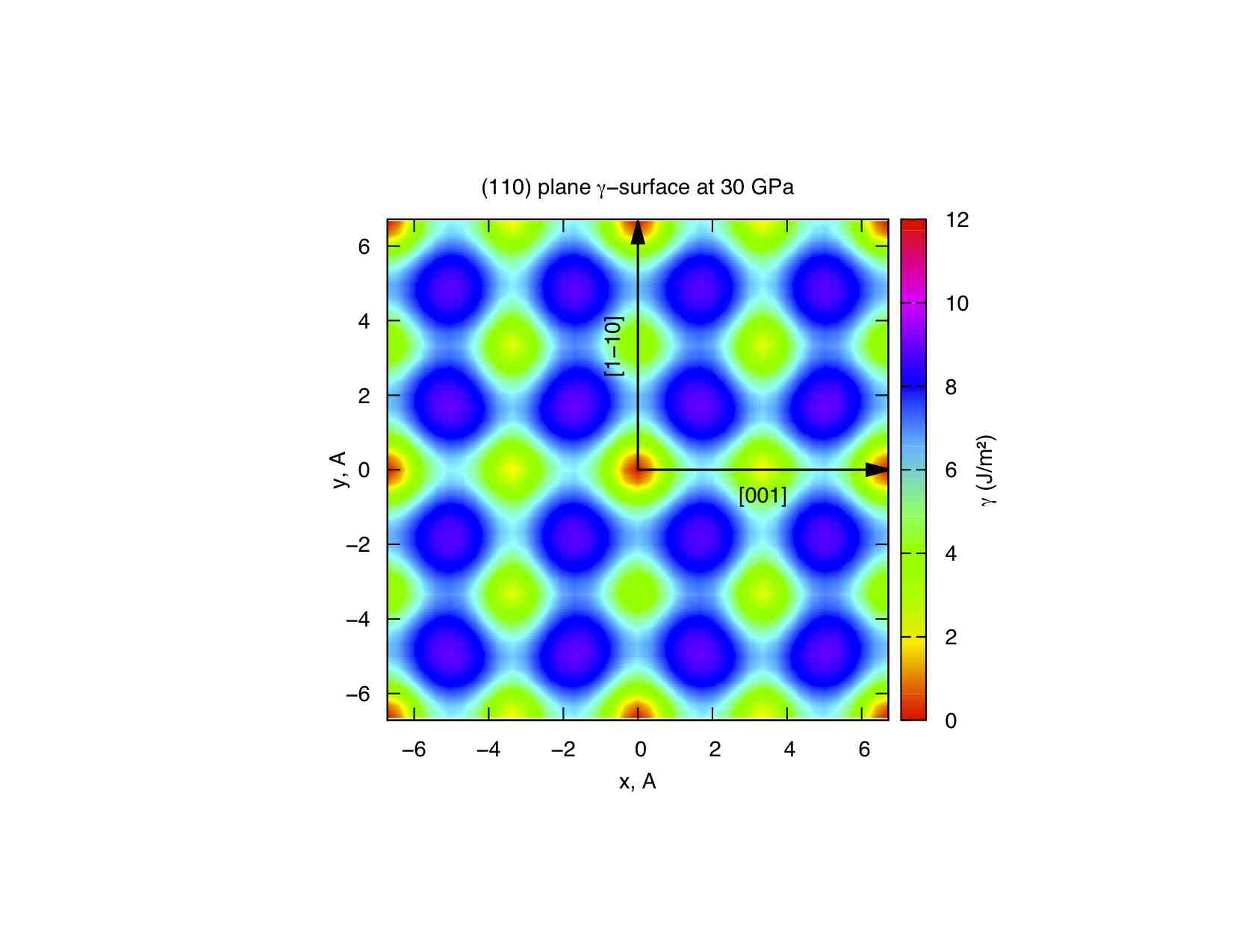V. Vitek, Phil. Mag., 18 (1968), pp. 773–786

 A. R. Oganov, J. P. Brodholt and G. D. Price, Physics of the Earth and Planetary Interiors 122 (200) p. 277